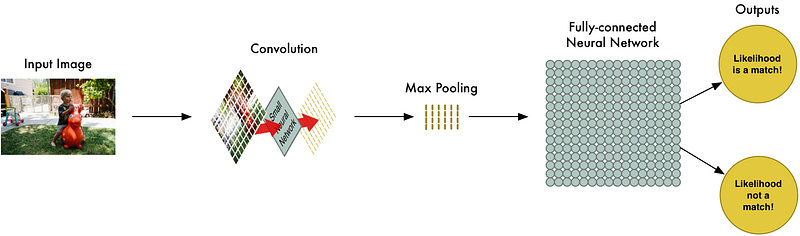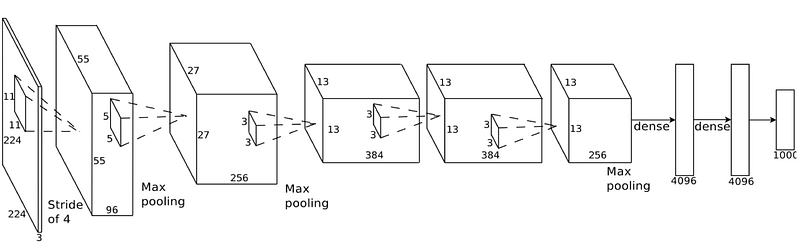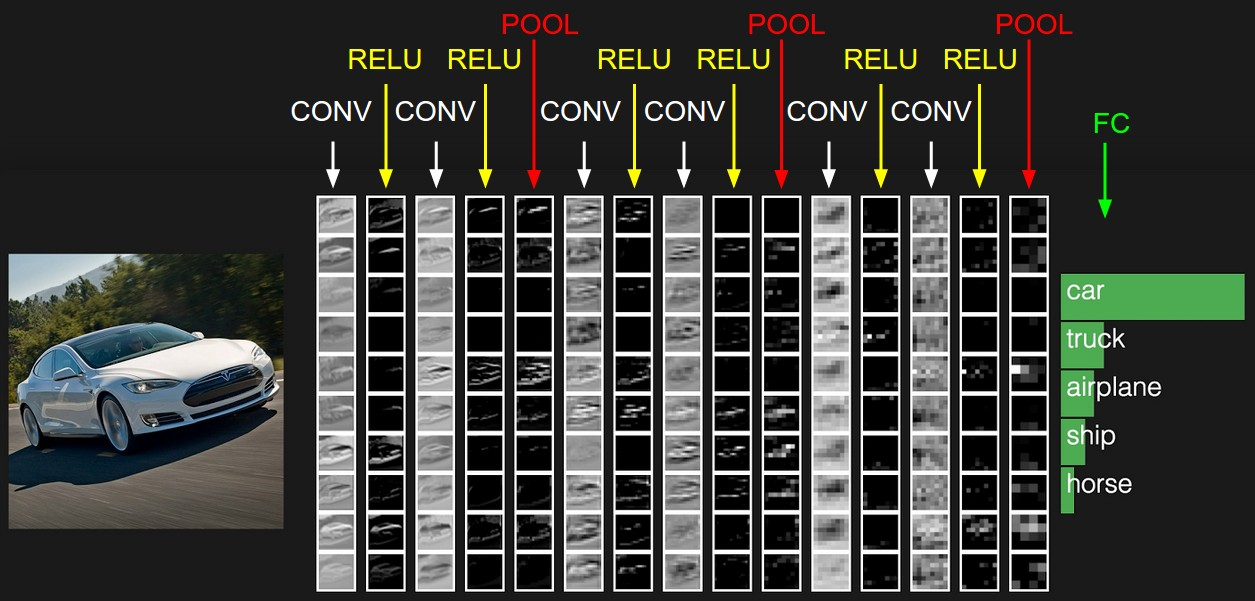Convolutional Neural Network

Convolutional Neural Networks (CNNs, or ConvNets) is a class of deep, feedforward artificial neural networks, commonly used to analyze visual imagery.Principles of a convolutional neural network

Composed of multiple layers with different types of operations, CNNs primarily rely upon the operation of convolution to recognize patterns.Diagram of a deep convolutional network

“…if all neurons in a single depth slice are using the same weight vector, then the forward pass of the CONV layer can in each depth slice be computed as a convolution of the neuron’s weights with the input volume (Hence the name: Convolutional Layer). This is why it is common to refer to the sets of weights as a filter (or a kernel), that is convolved with the input.”

-Stanford course CS231n: Convolutional Neural Networks for Visual Recognition.Activation schematic of a convolutional network

Read the detail post and course on computer vision and convolutional neural networks for more details about the architecture and evolutions of CNN algorithms.

Read more on Adam Geitgey’s article on CNNsStanford CS231n Github and Wikipedia

« Back to Glossary Index